## Proof That the Dot Product of a Vector With Itself Via a Skew Symmetric Matrix Is Zero

Theorem

If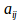is skew symmetric then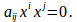Also ifthen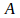is skew symmetric.

Proof

Sinceis skew symmetric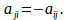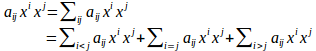If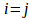then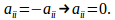If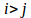then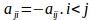so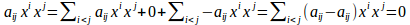Conversely, suppose.

Let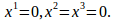Then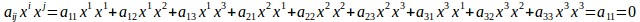Similarly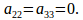Now take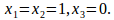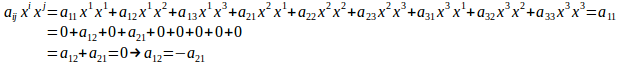Similarly

$a_{ij}=-a_{ji}, \: i,j=1,2,3.$